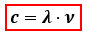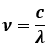Chemistry Practice Problems Bohr and Balmer Equations Practice Problems Solution: What wavelength of light will be required to remov...

🤓 Based on our data, we think this question is relevant for Professor Bojan's class at PSU.

# Solution: What wavelength of light will be required to remove an electron from the n = 3 shell of a hydrogen atom?

###### Problem

What wavelength of light will be required to remove an electron from the n = 3 shell of a hydrogen atom?

###### Solution

The wavelength of the light required to remove the electron from the n = 3 shell can be calculated using the speed of light:c = speed of light = 3.0x108 m/s
λ = wavelength, in m

Since we don’t have frequency, we can rearrange the equation:Recall the energy equation:View Complete Written Solution

Bohr and Balmer Equations

Bohr and Balmer Equations

#### Q. Using Bohr's equation for the energy levels of the electron in the hydrogen atom, determine the energy (J) of an electron in the n = 4 level.a) -5.45 ...

Solved • Tue Mar 15 2016 13:54:23 GMT-0400 (EDT)

Bohr and Balmer Equations

#### Q. What is the energy of the light emitted from a hydrogen atom as it relaxes from  the n = 5 to n = 3 excited states?                   What ...

Solved • Wed Feb 24 2016 16:04:40 GMT-0500 (EST)

Bohr and Balmer Equations

#### Q. A hydrogen atom emits light in an electronic transition going from n=4 to n=2 lower shell. 1.) What is the frequency of this light?  2.) What waveleng...

Solved • Wed Feb 24 2016 13:29:39 GMT-0500 (EST)

Bohr and Balmer Equations

#### Q. Atoms with one electron can be modeled with an equation similar to the Bohr equation:          Ea = -2.179x10-18 J (Z2/n2) In the equation, Z is the...

Solved • Mon Feb 22 2016 08:57:04 GMT-0500 (EST)